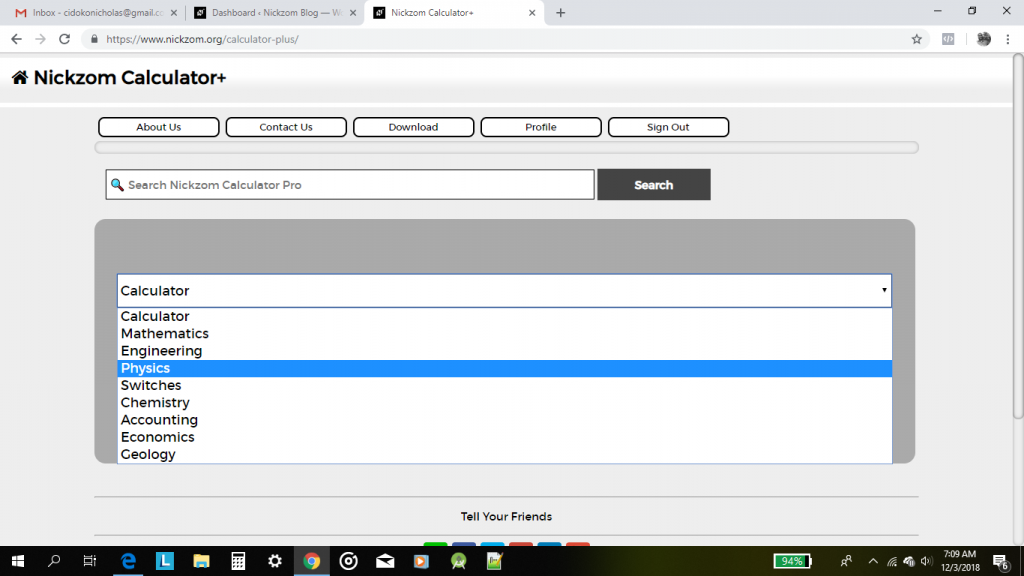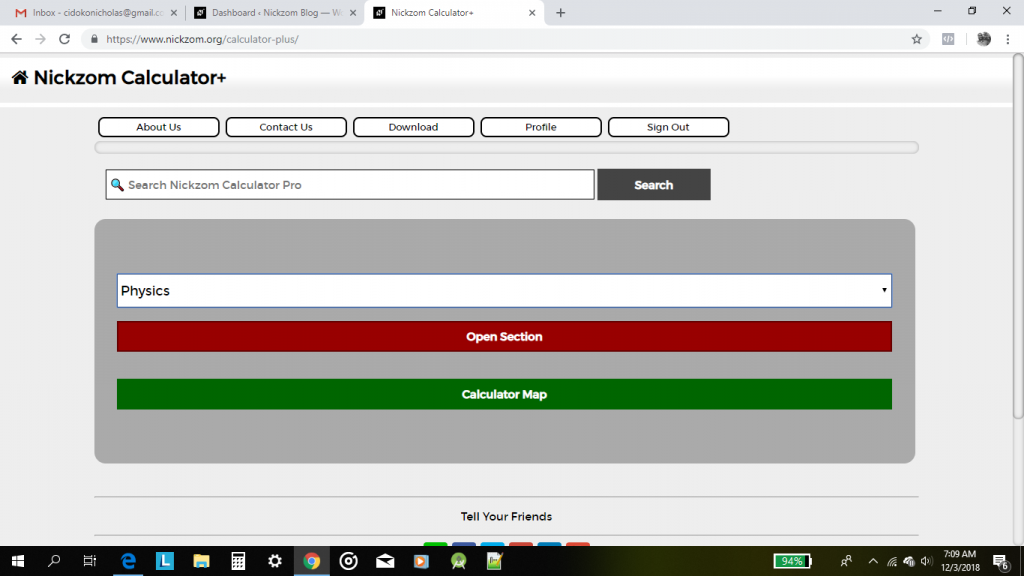## Nickzom Calculator Calculates the Total Time of Flight and Time of Flight at Maximum Height in Projectile (Physics)

I am not hear to start explaining to you the meaning and application of time of flight. I believe you are here to know how the calculator encyclopedia calculates these parameters.

Nickzom Calculator requires three parameters to get the answer for time of flight and these parameters are:

• Acceleration due to Gravity
• Initial Velocity
• Angle of Projection

Let’s first get the workings and answer for time of flight at maximum height where the acceleration due to gravity is 10 m/s2, initial velocity is 20 m/s and angle of projection is 30°.

First and Foremost, Select Physics from the home page and click on Open SectionIn the Physics section, Click on Projectile